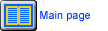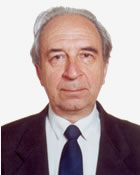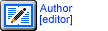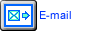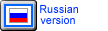Theoretical Dialectical Journal: Physics-Mathematics-Logic-Philosophy Journal represents Theoretical Dialectics in operation For the first time in science the bases mathematical dialectics with the application to fundamental questions of physics and mathematics are stated on the basis of a dialectical binary numerical wave field, which opportunities considerably surpass existing traditional mathematical methods.With its outstanding achievements in exact sciences, especially physics, the 20th century will rightfully take a special place in the history of human kind. However, at present physics increasingly lags behind general progress, raising serious doubts about some of its basic concepts. To some extent, this is also true of mathematics. Therefore, it seems to be necessary to revise the basic axioms of physics and mathematics and to analyze them from the general standpoint of Dialectics (Dialectical Logic and Dialectical Philosophy).     In this journal the author have concentrated on general problems of physical axioms, which are considered within Hegelian dialectics, including logic, philosophy and mathematics.     As is known, the main characteristic feature of dialectics is a juncture of the material and ideal Worlds, which is the most important contradiction of the Universe. This contradiction joins all the other contradictions of the Universe. Of all philosophic systems, dialectics alone expresses the objective contradictions most completely by considering them as a basis for everything that exists in the Universe. Translating the concept of contradictions and noncontradictions into the language of mathematics, the author have constructed a mathematical description based on the mathematics of the material-ideal numerical field, which allows sufficiently accurate expression of the laws of dialectics in mathematical form and, proceeding from them, a description of axioms of physics, and mathematics.      All subsequent articles are written in language of dialectics and a dialectic binary numerical wave field, and assume knowledge of articles published here: [Issue 1.Mathematical expression of the main categories of Dialectical Philosophy and Logic]